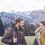# Which option is correct?If $xyz=-1$, then the value of $\sqrt [ 4 ]{ \left( xyz \right)^4 }$ is

Option 1 : 1

Why?

Because

$\Large{ \sqrt [ 4 ]{ \left( xyz \right)^4} =\sqrt [ 4 ]{ \left( -1 \right) ^{ 4 } } =\sqrt [ 4 ]{ 1 } =1}$

Option 2 : -1

Why?

Because

$\Large{\sqrt [ 4 ]{ \left( xyz \right) ^{ 4 } }=xyz=-1}$

Option 3 : $i$

Why?

Because

$\Large{\sqrt [ 4 ]{ \left( xyz \right) ^{ 4 } } =\quad \sqrt [ 4 ]{ \left( xyz \right) ^{ 3 } } \times \sqrt [ 4 ]{ xyz } =\sqrt { i } \times \sqrt { i } =i}$

Explanation requiredNote by Department 8
5 years, 6 months ago

This discussion board is a place to discuss our Daily Challenges and the math and science related to those challenges. Explanations are more than just a solution — they should explain the steps and thinking strategies that you used to obtain the solution. Comments should further the discussion of math and science.

When posting on Brilliant:

• Use the emojis to react to an explanation, whether you're congratulating a job well done , or just really confused .
• Ask specific questions about the challenge or the steps in somebody's explanation. Well-posed questions can add a lot to the discussion, but posting "I don't understand!" doesn't help anyone.
• Try to contribute something new to the discussion, whether it is an extension, generalization or other idea related to the challenge.

MarkdownAppears as
*italics* or _italics_ italics
**bold** or __bold__ bold
- bulleted- list
• bulleted
• list
1. numbered2. list
1. numbered
2. list
Note: you must add a full line of space before and after lists for them to show up correctly
paragraph 1paragraph 2

paragraph 1

paragraph 2

[example link](https://brilliant.org)example link
> This is a quote
This is a quote
    # I indented these lines
# 4 spaces, and now they show
# up as a code block.

print "hello world"
# I indented these lines
# 4 spaces, and now they show
# up as a code block.

print "hello world"
MathAppears as
Remember to wrap math in $$ ... $$ or $ ... $ to ensure proper formatting.
2 \times 3 $2 \times 3$
2^{34} $2^{34}$
a_{i-1} $a_{i-1}$
\frac{2}{3} $\frac{2}{3}$
\sqrt{2} $\sqrt{2}$
\sum_{i=1}^3 $\sum_{i=1}^3$
\sin \theta $\sin \theta$
\boxed{123} $\boxed{123}$

Sort by:

Ex. $(\sqrt{-1})^2 \neq \sqrt{(-1)^2}$
LHS : $(\sqrt{-1})^2 = -1$
RHS : $\sqrt{(-1)^2} = |-1| = 1$

I think all the options are correct. Given equation has 4 roots.i.e $1 , -1 , \iota , -\iota$

- 5 years, 6 months ago

Option $0!$

- 5 years, 6 months ago

Option 1, because the property that (a)^(mn)=a^m*a^n is only preserved for real numbers.

- 5 years, 6 months ago

Option 1. First condition: xyz= -1

- 5 years, 6 months ago

let x = xyz^4 so x will have roots ie i,-1,1,one more

- 5 years, 6 months ago

Option 1

- 5 years, 6 months ago

Explanation required

- 5 years, 6 months ago

(x^2n)^(1/2n)=|x|

- 5 years, 6 months ago

Nice

- 5 years, 6 months ago

Ya correct explanation.....its option 1

- 5 years, 6 months ago

option 1 guaranteed .option 2-no because the root sign stands for only positive values and option 3 -no because u cant do it for unreal numbers

- 5 years, 6 months ago